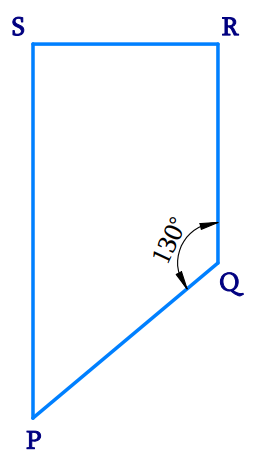# Ex.3.3 Q12 Understanding Quadrilaterals Solution-Ncert Maths Class 8

Go back to  'Ex.3.3'

## Question

Find the measure of $$\angle {\rm{P }}$$ and $$\angle {\rm{S}}$$ if $$SP$$ is parallel to $$RQ$$ in Fig .

(If you find $${\rm{m}}\angle {\rm{R}}$$, is there more than one method to find $${\rm{m}}\angle {\rm{P}}\,?$$Video Solution
Ex 3.3 | Question 12

## Text Solution

What is Known?

What is Unknown?

Find $${\rm{m}}\angle P\,{\rm{ and}\, {m}}\angle S$$

Reasoning:

Sum of the measures of all the interior angles of a quadrilateral is $$360^\circ$$.

Steps:Given $$SP$$ is parallel $$RQ$$ and $$SR$$ is the traversal drawn to these lines. Hence,

\begin{align}\angle \text{S}+\angle \text{R}&={{180}^{\text{o}}}\\\angle \text{S}+{{90}^{\circ}}&={{180}^{\circ}} \\\angle \text{S}&={{180}^{\circ}}-{{90}^{\circ}} \\\angle \text{S}&={{90}^{\circ}} \\ \end{align}

Using the angle sum property of a quadrilateral,

\begin{align}\angle \text{S}+\angle \text{P}+\angle \text{Q}+\angle \text{R}&={{360}^{\text{o}}} \\{{90}^{\text{o}}}+\angle \text{P}+{{130}^{\text{o}}}+{{90}^{\text{o}}}&={{360}^{\text{o}}} \\\angle \text{P}+{{310}^{\text{o}}}&={{360}^{\text{o}}} \\\angle \text{P}&\!\!=\!{{360}^{\text{o}}}\!\!-\!\!{{310}^{\text{o}}} \\\angle \text{P}&={{50}^{\text{o}}} \\\end{align}

Learn from the best math teachers and top your exams

• Live one on one classroom and doubt clearing
• Practice worksheets in and after class for conceptual clarity
• Personalized curriculum to keep up with school